# Microbiology: Chapter 2 Flashcards

Set Details Share
created 7 years ago by Lazybones
5,328 views
updated 7 years ago by Lazybones
Subjects:
science, life sciences, biology, microbiology
Page to share:
Embed this setcancel
COPY
code changes based on your size selection
Size:
X

1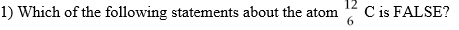A) It has 6 protons in its nucleus.

B) It has 12 neutrons in its nucleus.

C) It has 6 electrons orbiting the nucleus.

D) Its atomic number is 6.

E) Its atomic weight is 12.

2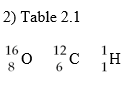Using the information in Table 2.1, calculate the molecular weight of ethanol, C2H5OH.

A) 96

B) 46

C) 34

D) 33

E) The answer cannot be determined.

3

3) Antacids neutralize acid by the following reaction. Identify the salt in the following equation:

Mg(OH)2 + 2HCl → MgCl2 + H2O

A) Mg(OH)2

B) HCl

C) MgCl2

D) H2O

E) None of the answers is correct.

4

4) Which of the following statements is FALSE?

A) Salts readily dissolve in water.

B) Water molecules are formed by hydrolysis.

C) Water freezes from the top down.

D) Water is formed as a part of a dehydration synthesis reaction.

E) Water is a polar molecule.

5

5) Which of the following is the type of bond holding K+ and I- ions in KI?

A) ionic bond

B) covalent bond

C) hydrogen bond

6

6) Which of the following is the type of bond between molecules of water in a beaker of water?

A) ionic bond

B) covalent bond

C) hydrogen bond

7

7) What is the type of bond holding hydrogen and oxygen atoms together in a single H2O molecule?

A) ionic bond

B) covalent bond

C) hydrogen bond

8

8) Identify the following reaction: Glucose + Fructose → Sucrose + Water

A) dehydration synthesis reaction

B) hydrolysis reaction

C) exchange reaction

D) reversible reaction

E) ionic reaction

9

9) Identify the following reaction: Lactose + H2O → Glucose + Galactose

A) dehydration synthesis reaction

B) hydrolysis reaction

C) exchange reaction

D) reversible reaction

E) ionic reaction

10

10) Identify the following reaction: HCl + NaHCO3 → NaCl + H2CO3

A) dehydration synthesis reaction

B) hydrolysis reaction

C) exchange reaction

D) reversible reaction

E) ionic reaction

11

11) Identify the following reaction: NH4OH ⇌ NH3 + H2O

A) dehydration synthesis reaction

B) hydrolysis reaction

C) exchange reaction

D) reversible reaction

E) ionic reaction

12

12) Which type of molecule contains the alcohol glycerol?

A) carbohydrate

B) phospholipids

C) DNA

D) protein

13

13) Which type of molecule is composed of (CH2O) units?

A) carbohydrate

B) lipid

C) nucleic acid

D) protein

14

14) Which type of molecule contains -NH2 (amino) groups?

A) carbohydrate

B) triglycerides

C) nucleic acid

D) protein

15

15) Which type of molecule NEVER contains a phosphate group?

A) triglycerides

B) phospholipid

C) nucleic acid

D) ATP

16

16) Based upon the valence numbers of the elements magnesium (2) and hydrogen (1), predict how many covalent bonds would form between these atoms to achieve the full complement of electrons in their outermost energy shells.

A) one

B) two

C) three

D) four

17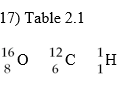Using the information in Table 2.1, calculate the number of moles in 92 grams of ethanol,

C2H5OH.

A) 1

B) 2

C) 3

D) 4

E) The answer cannot be determined.

18

18) Which of the following statements regarding protein structure is FALSE?

A) The primary structure is formed by covalent bonding between amino acid subunits.

B) Secondary structures are formed only from hydrogen bonds.

C) Tertiary structures are formed only from covalent bonds.

D) Quaternary structures involved multiple polypeptides.

19

19) Which of the following pairs is mismatched?

A) NaOH ⇌ Na++ OH-— base

B) HF ⇌ H++ F-— acid

C) MgSO4⇌ Mg2++ SO42- — salt

D) KH2PO4⇌ K++ H2PO4- — acid

E) H2SO4⇌ 2H++ SO42- — acid

20

20) Table 2.2

NaOH ⇌ Na+ + OH- — base

HF ⇌ H+ + F- - acid

MgSO4 ⇌ Mg2+ + SO42- — salt

KH2PO4 ⇌ K+ + H2PO4- — acid

H2SO4 ⇌ 2H+ + SO42- — salt

Which of the following statements about the reactions in Table 2.2 is FALSE?

A) They are exchange reactions.

B) They are ionization reactions.

C) They occur when the reactants are dissolved in water.

D) They are dissociation reactions.

E) They are reversible reactions.

21

21) What is the type of weak bond between the hydrogen of one molecule and the nitrogen of another molecule, where the two don't actively share an electron?

A) ionic bond

B) covalent bond

C) hydrogen bond

D) disulfide bond

E) hydrophobic bond

22

22) What is the type of strong chemical bond between carbon, hydrogen, and oxygen atoms in a single organic molecule?

A) ionic bond

B) covalent bond

C) hydrogen bond

23

23) What is the type of bond between ions in salt?

A) ionic bond

B) covalent bond

C) hydrogen bond

24

24) A scientist wants to perform a test that will indicate whether a nucleic acid sample is composed of RNA or DNA. Testing for the presence of which of the following is most appropriate in this situation?

A) phosphate

B) nitrogen

C) guanine

D) uracil

E) thymine

25

25) Structurally, ATP is most like which type of molecule?

A) carbohydrate

B) lipid

C) protein

D) nucleic acid

26

26) What do genes consist of?

A) carbohydrates

B) lipids

C) proteins

D) nucleic acids

27

27) Which molecule is composed of a chain of amino acids?

A) carbohydrate

B) lipid

C) protein

D) nucleic acid

28

28) Which are the primary molecules making up plasma membranes in cells?

A) carbohydrates

B) lipids

C) proteins

D) nucleic acids

29

29) The antimicrobial drug imidazole inhibits sterol synthesis. This would most likely interfere with

A) bacterial cell walls.

B) fungal cell walls.

C) eukaryotic plasma membranes.

D) prokaryotic plasma membranes.

E) genes.

30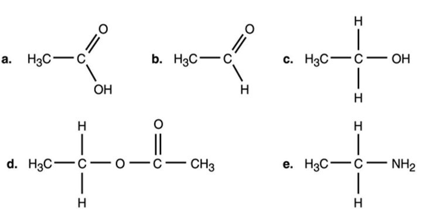30) In Figure 2.1, which is an alcohol?

A) a

B) b

C) c

D) d

E) e

3131) Which compound in Figure 2.1 is an ester?

1. A) a
2. B) b
3. C) c
4. D) d
5. E) e

3232) Which compound in Figure 2.1 is an organic acid?

1. A) a
2. B) b
3. C) c
4. D) d
5. E) e

33

33) Most amino acids found in cells demonstrate what type of chirality?

A) L-isomers

B) D-isomers

C) C-isomers

D) B-isomers

E) A-isomers

34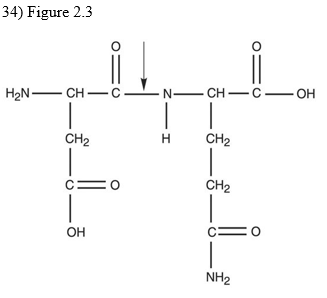What kind of bond is at the arrow in Figure 2.3?

A) disulfide bridge

B) double covalent bond

C) hydrogen bond

D) ionic bond

E) peptide bond

35

35) An E. coli culture that has been growing at 37°C is moved to 25°C. Which of the following changes must be made in its plasma membrane to help it cope with the decrease in temperature?

A) The number of phosphate groups must increase.

B) The viscosity must increase.

C) The number of saturated chains must increase.

D) The number of unsaturated chains must increase.

E) No changes are necessary

36

36) Radioisotopes are frequently used to label molecules in a cell. The fate of atoms and molecules in a cell can then be followed. Assume Saccharomyces cerevisiae is grown in a nutrient medium containing the radioisotope 35S. After a 48-hour incubation, the 35S would most likely be found in the S. cerevisiae's

A) carbohydrates.

B) nucleic acids.

C) water.

D) lipids.

E) proteins.

37

37) Radioisotopes are frequently used to label molecules in a cell. The fate of atoms and molecules in a cell can then be followed. Assume Saccharomyces cerevisiae is grown in a nutrient medium containing the radioisotope 32P. After a 48-hour incubation, the majority of the 32P would be found in the S. cerevisiae's

A) plasma membrane.

B) cell wall.

C) water.

D) proteins.

E) carbohydrates.

38

38) Starch, dextran, glycogen, and cellulose are polymers of

A) amino acids.

B) glucose.

C) fatty acids.

D) nucleic acids.

E) acids.

39

39) Which of the following is a base?

A) C2H5OCOOH → H++ C2H5OCOO-

B) C2H5OH

C) NaOH → Na++ OH-

D) H2O → H++ OH-

E) H2CO

40

40) Two glucose molecules are combined to make a maltose molecule. What is the chemical formula for maltose?

A) C3H6O3

B) C6H12O6

C) C12H24O12

D) C12H22O11

E) C12H23O10

41

41) If an amino acid contained a hydrocarbon (a group of multiple carbons and hydrogens linked together) as its side group, in which of the following categories could it be appropriately designated?

A) hydrophilic

B) polar

C) nonpolar

D) basic

E) acidic